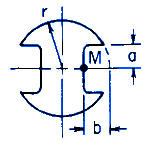Related Resources: calculators

### Shaft with Two Keyways Torque Applied Deformation and Stress Equations and Calculator

Beam Deflection and Stress Equation and Calculators

Torque Applied Deformation and Stress of a Shaft with Two Keyways Equations and CalculatorALL calculators require a Premium Membership

Preview

Torque Applied Deformation and Stress of a Shaft with Keyway Section Calculator

K = 2Cr4

Varies with b / r as follows

For:

0   ≤   b / r ≤   0.5Where 0.3   ≤   a / b   ≤   1.5Angle of Twist under applied Torque Moment

θ = ( T L ) / ( K G )

Shear Stress Maximum

At M:Where B varies with b / r as follows:for 0.5 ≤ a / b ≤ 1.5Where:

θ = angle of twist (radians),
T = Twisting or torque moment force-length, (in-lbs, N-mm),
L = Length of Section (in, mm),
h = Length as Indicated (in, mm),
a,b = Distance as shown (in, mm),
τ = Unit shear stress force / area (lbs/in2, N/mm2),
G = Modulus of rigidity force / area (lbs/in2, N/mm2),
K = Polar Moment of Inertia (in4, mm4) for section

Reference:
Roarks Formulas for Stress and Strain, 7th Edition, Table 10.1 Formulas for torsional deformation and stress.

Related:

The Shaft Design Book (Design Charts and Calculations for Torsional Properties of Non-Circular Shafts)